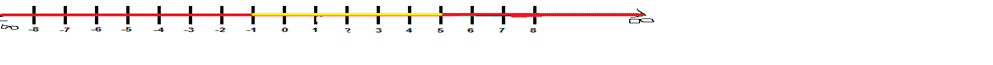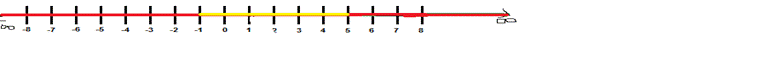# To Express: The inequalities in terms of the interval. − 1 ≺ x ≤ 5 .### Precalculus: Mathematics for Calcu...

6th Edition
Stewart + 5 others
Publisher: Cengage Learning
ISBN: 9780840068071### Precalculus: Mathematics for Calcu...

6th Edition
Stewart + 5 others
Publisher: Cengage Learning
ISBN: 9780840068071

#### Solutions

Chapter 1, Problem 8RE
To determine

## To Express: The inequalities in terms of the interval. −1≺x≤5 .

Expert Solution

In this interval 1x5 , x is a member of family of real numbers such that x is a number of the interval {xR\1x5} .### Explanation of Solution

Given information:

The inequality 1x5 ..

Formula used:

The notation of number line shows the given interval (] shows that open and a closed interval.

Calculation:

It is provided that the notation (1,5] . ,shows that the real numbers are here starting form less then -1 and ended at 5.

So the interval is , {xR\1x5} .

This is the written statement of this notation and here x is a member of family of real numbers such that x is a number of the interval (1,5] .

Also this equation is written as: {xR\x(1,5]} .

The notation of these inequalities are here:Thus, this inequality is an open and closed interval , it means it starts form less then -1 and ended to 5.

### Have a homework question?

Subscribe to bartleby learn! Ask subject matter experts 30 homework questions each month. Plus, you’ll have access to millions of step-by-step textbook answers!